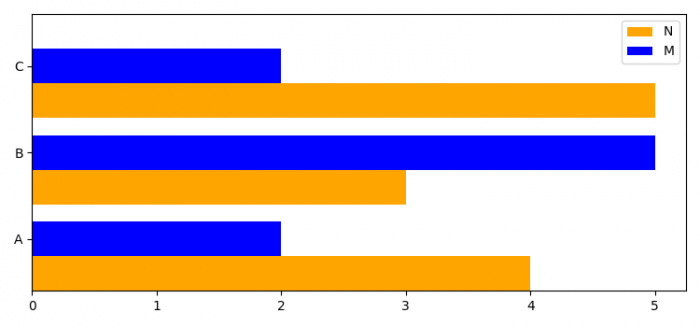# How to plot multiple horizontal bars in one chart with matplotlib?

To plot multiple horizontal bars in one chart with matplotlib, we can take the following steps −

## Steps

• Import the libraries pandas, matplotlib, and numpy.

• Set the figure size and adjust the padding between and around the subplots.

• Create an array for horizontal bar's position.

• Initialize a variable width for bar's width.

• Create a horizontal bar plot.

• Set Y-axis ticks and tick labels with some limit.

• Place a legend on the plot at the upper right location.

• To display the figure, use show() method.

## Example

import pandas
import matplotlib.pyplot as plt
import numpy as np

# Set the figure size
plt.rcParams["figure.figsize"] = [7.50, 3.50]
plt.rcParams["figure.autolayout"] = True

# Array for horizontal bar's position
ind = np.array([0, 1, 2])

# Bar's width
width = 0.4

fig, ax = plt.subplots()

# Horizontal bar plot
ax.barh(ind, np.array([4, 3, 5]), width, color='orange', label='N')
ax.barh(ind + width, np.array([2, 5, 2]), width, color='blue', label='M')

# Set Y-axis ticks and ticklabels
ax.set(yticks=ind + width, yticklabels=np.array(['A', 'B', 'C']),
ylim=[2*width - 1, len(ind)])

# Legend at the upper right corner
ax.legend(loc='upper right')

# Display the plot
plt.show()

## Output

It will produce the following output −